# SUMPRODUCT Function

The SUMPRODUCT function returns the sum of the product of one or more arrays of values.
This means that the corresponding items in the arrays are multiplied together and then added up.
All the cell range arguments must be the same size and it is not possible to include whole columns (unless you are using Excel 2007).

The following two examples show this function in its most simple form.
The formula in cell "B7" is multiplying the two columns of numbers and then adding them up.
This function is equivalent to the formulas in cells "B7" and "B8".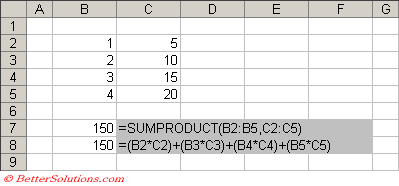Any text that may be included in your arrays will be ignored.
The following example shows what the result is when one of the arrays contains some text.
In this case the product of anything multiplied by text is zero.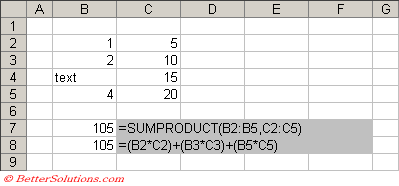### Removing a Subtotal Column

The following table contains information about how many people were working on a particular day and how long they were working for.
Lets suppose we want the total number of hours worked in this table.
We could obtain a subtotal for each day (column "F") and then total these cells (cell "F8") or we could just use the this function.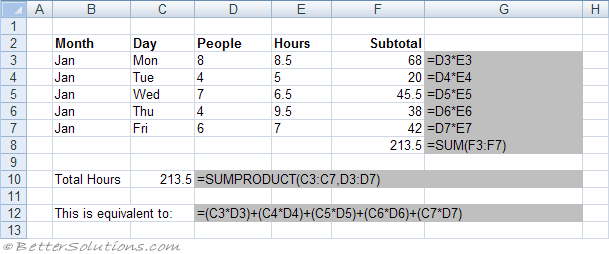This function takes cell ranges (or arrays) as its arguments and multiplies the corresponding elements together and then adds all the results.
The function used in cell "C12" is equivalent to the formula displayed in cell "D14".

### Conditional Counting

This function can be used for counting based on one or multiple conditions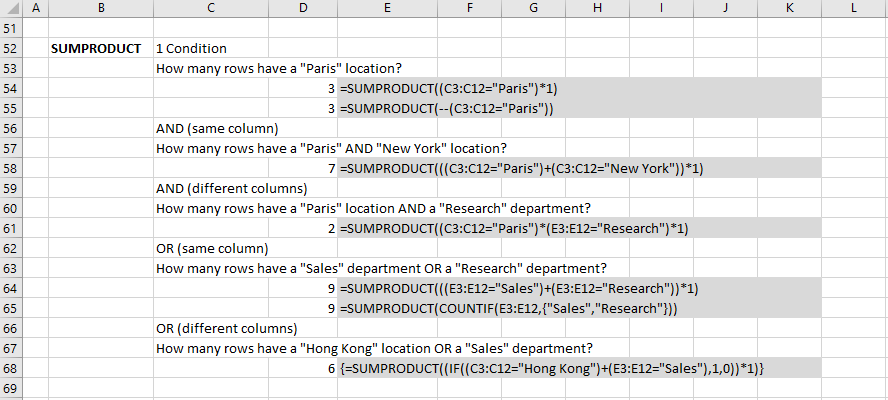### Conditional Summing

This function can be used for summing based on one or multiple conditions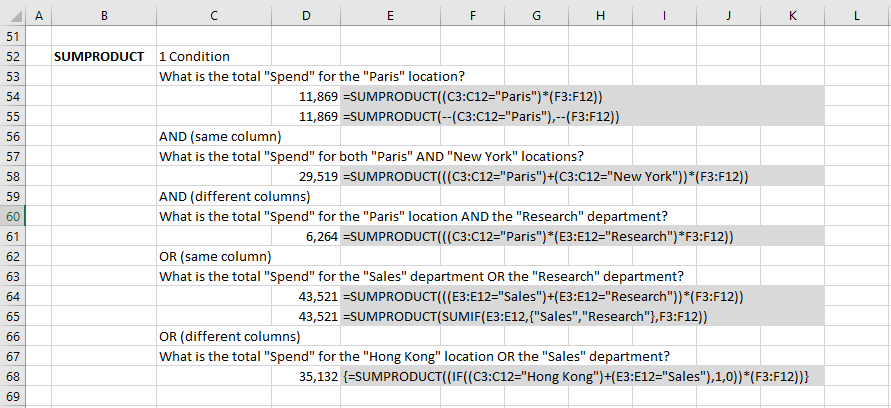### Referencing and Updating from Closed Workbooks

This function can reference and update from closed workbooks.